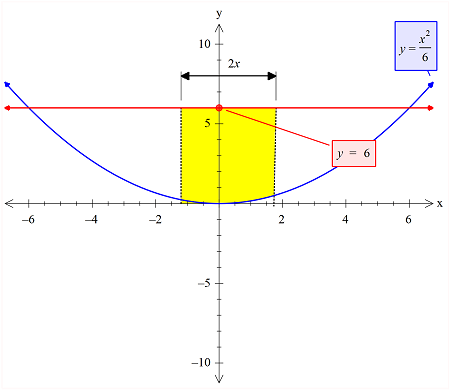# A solid is generated by revolving the region bounded by the following about the y-axis. { y =...

## Question:

A solid is generated by revolving the region bounded by the following about the y-axis. {eq}y = \frac{1}{6} x^2 , {/eq} y = 6 A hole, centered along the axis of revolution, is drilled through this solid so that one-fourth of the volume is removed.

Find the diameter of the hole. (Round your answer to three decimal places.)

## Definite Integrals::

When the volume of the solid is given, then we can find the volume of the another solid that is taken out from it under some given conditions, with the help of the definite integral set up. The limits of integration are important and should be as per the given condition.

The hole that is drilled is shown in the figure in yellow color. Note that this is the cross-sectional area that is represented here.The diameter of the hole is:

d=2x

and the height is:

h=6

So the volume of this cylinder is:

{eq}V_1= 6\pi x^2 {/eq}

Now, this volume is one-fourth of the total volume that is found by rotation of the common region of the curve as shown.

The total volume is given as:

{eq}V=\int_{0}^{6}\pi x^2 dy\\ =\int_{0}^{6}\pi 6y dy~~~~~~~~~~~~\left [ \because x^2=6y \right ]\\ =6\pi \left[\frac{y^2}{2}\right]^6_0\\ =108\pi {/eq}

So now we have:

{eq}6\pi x^2= \frac{108\pi }{4}\\ => x=\frac{3\sqrt{2}}{2}\\ {/eq}

So the diameter of the hole is:

{eq}2x= 3\sqrt{2}\\ {/eq}